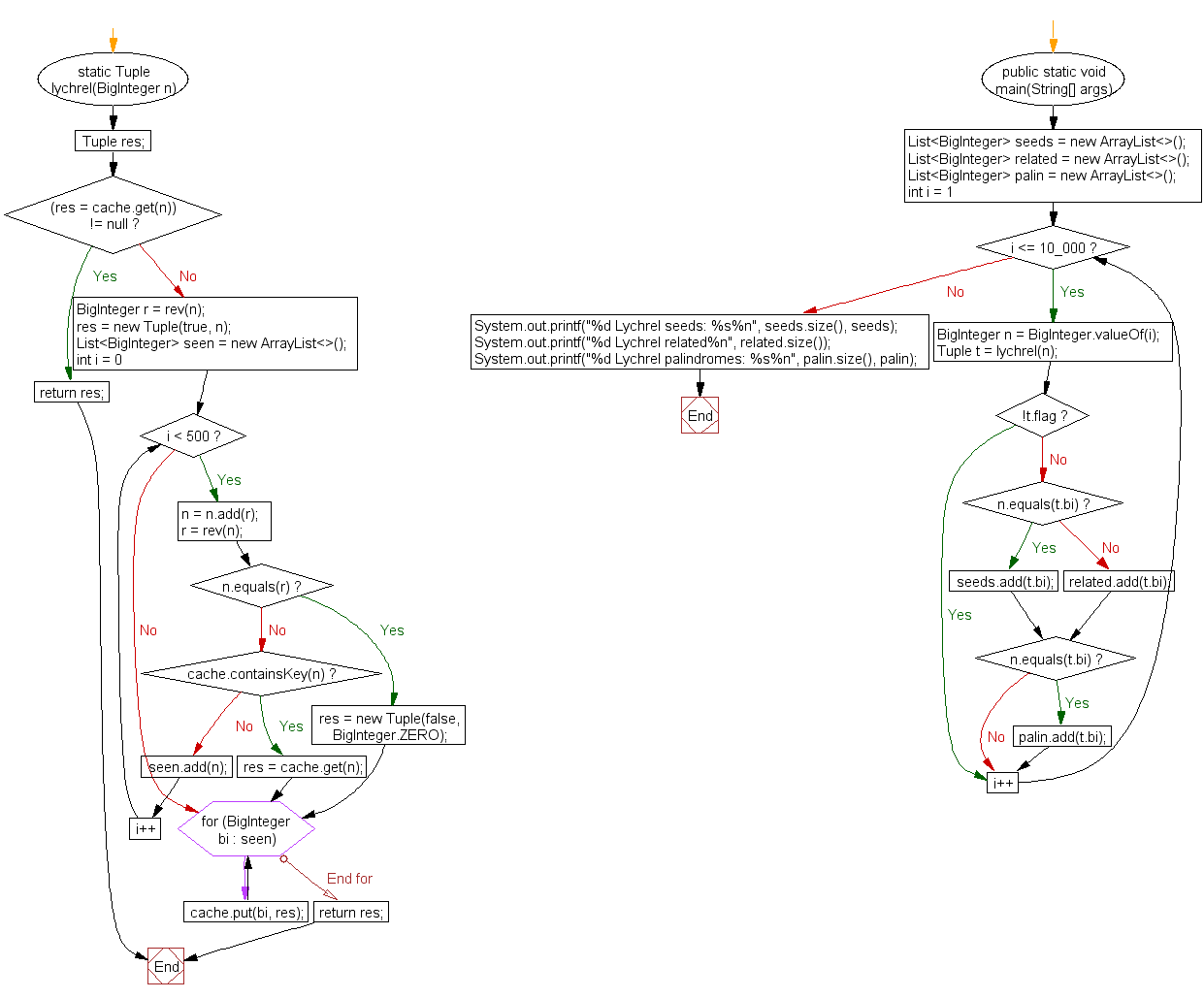﻿ Java exercises: Find the number of seed Lychrel number candidates and related numbers for n in the range 1..10000 inclusive - w3resource# Java Exercises: Find the number of seed Lychrel number candidates and related numbers for n in the range 1..10000 inclusive.

## Java Numbers: Exercise-5 with Solution

Write a Java program to find the number of seed Lychrel number candidates and related numbers for n in the range 1..10000 inclusive. (With that iteration limit of 500).

A Lychrel number is a natural number that cannot form a palindrome through the iterative process of repeatedly reversing its digits and adding the resulting numbers. This process is sometimes called the 196-algorithm, after the most famous number associated with the process.
The first few Lychrel numbers are 196, 295, 394, 493, 592, 689, 691, 788, 790, 879, 887, ... .

Pictorial Presentation:Sample Solution:

Java Code:

``````// https://rosettacode.org/
import java.math.BigInteger;
import java.util.*;
public class Example5 {
static Map<BigInteger, Tuple> cache = new HashMap<>();
static class Tuple {
final Boolean flag;
final BigInteger bi;

Tuple(boolean f, BigInteger b) {
flag = f;
bi = b;
}
}
static BigInteger rev(BigInteger bi) {
String s = new StringBuilder(bi.toString()).reverse().toString();
return new BigInteger(s);
}
static Tuple lychrel(BigInteger n) {
Tuple res;
if ((res = cache.get(n)) != null)
return res;

BigInteger r = rev(n);
res = new Tuple(true, n);
List<BigInteger> seen = new ArrayList<>();
for (int i = 0; i < 500; i++) {
r = rev(n);

if (n.equals(r)) {
res = new Tuple(false, BigInteger.ZERO);
break;
}
if (cache.containsKey(n)) {
res = cache.get(n);
break;
}
}
for (BigInteger bi : seen)
cache.put(bi, res);
return res;
}
public static void main(String[] args) {
List<BigInteger> seeds = new ArrayList<>();
List<BigInteger> related = new ArrayList<>();
List<BigInteger> palin = new ArrayList<>();

for (int i = 1; i <= 10_000; i++) {
BigInteger n = BigInteger.valueOf(i);
Tuple t = lychrel(n);
if (!t.flag)
continue;
if (n.equals(t.bi))
else

if (n.equals(t.bi))
}
System.out.printf("%d Lychrel seeds: %s%n", seeds.size(), seeds);
System.out.printf("%d Lychrel related%n", related.size());
System.out.printf("%d Lychrel palindromes: %s%n", palin.size(), palin);
}
}
```
```

Sample Output:

```5 Lychrel seeds: [196, 879, 1997, 7059, 9999]
244 Lychrel related
5 Lychrel palindromes: [196, 879, 1997, 7059, 9999]
```

Flowchart:Java Code Editor:

What is the difficulty level of this exercise?

﻿

## Java: Tips of the Day

Array vs ArrayLists:

The main difference between these two is that an Array is of fixed size so once you have created an Array you cannot change it but the ArrayList is not of fixed size. You can create instances of ArrayLists without specifying its size. So if you create such instances of an ArrayList without specifying its size Java will create an instance of an ArrayList of default size.

Once an ArrayList is full it re-sizes itself. In fact, an ArrayList is internally supported by an array. So when an ArrayList is resized it will slow down its performance a bit as the contents of the old Array must be copied to a new Array.

At the same time, it's compulsory to specify the size of an Array directly or indirectly while creating it. And also Arrays can store both primitives and objects while ArrayLists only can store objects.

Ref: https://bit.ly/3o8L2KH